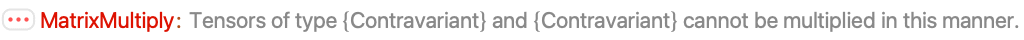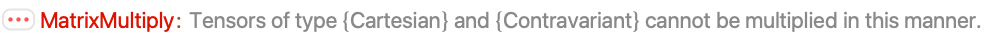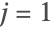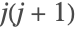# Inner Products and Matrix Multiplication

In quantum-mechanical matrix notation, the inner product and the action of operators on state vectors are implemented as matrix multiplication. The standard Dot product in Mathematica does not properly handle the inner product of vectors whose components are operators, because it tries to contract the last index of the first argument with the first index of the second argument.

The AtomicDensityMatrix package provides the function MatrixMultiply that takes into account the meaning of various tensor structures employed by the package when combining them. The package defines CenterDot to be equivalent to MatrixMultiply. CenterDot can be entered as ..

 MatrixMultiply[a,b] or a·b inner or outer product of spherical tensors, cartesian tensors, and operators TensorNorm[expr] norm of expr

Matrix multiplication.

 Matrix multiplication of a bra vector (covariant spherical tensor) with a ket vector (contravariant spherical tensor). The result is a scalar.
 Matrix multiplication in the reverse order produces an operator (the outer product).
 Matrix multiplication contracts an operator with a bra to its left, or a ket to its right.

Matrix multiplication is applied to each tensor of a tensor decomposition.

 Decomposition decomposes an operator into polarization moments.
 The sum of the inner products of each rank tensor with itself is equal to the square of the trace of the original operator.
 The matrix product of two Cartesian vectors is just the inner product.
 A matrix product involving a scalar reduces to simple multiplication.
 Multiplication of two contravariant or two covariant tensors is not allowed.Neither is multiplication on the wrong side of an operator.Or multiplication of a spherical with a Cartesian tensor.Matrix multiplication is recursively applied to tensor structures.

 The covariant spherical tensor components of the angular momentum operator for astate.
 When the inner product of the J operator and its dual are taken, the components are combined using matrix multiplication. The result is, as we expect,times the identity operator.
 This also works for the Cartesian components of the vector. Here are the Cartesian components of the angular-momentum operator.
 And the inner product with itself.

The norm of a tensor is the square root of the inner product of the tensor with its dual tensor. Cartesian vectors are equal to their dual, while the dual of a covariant spherical tensor is the contravariant tensor, and vice versa.

 A Cartesian vector and its norm.
 The corresponding spherical tensor and its norm.